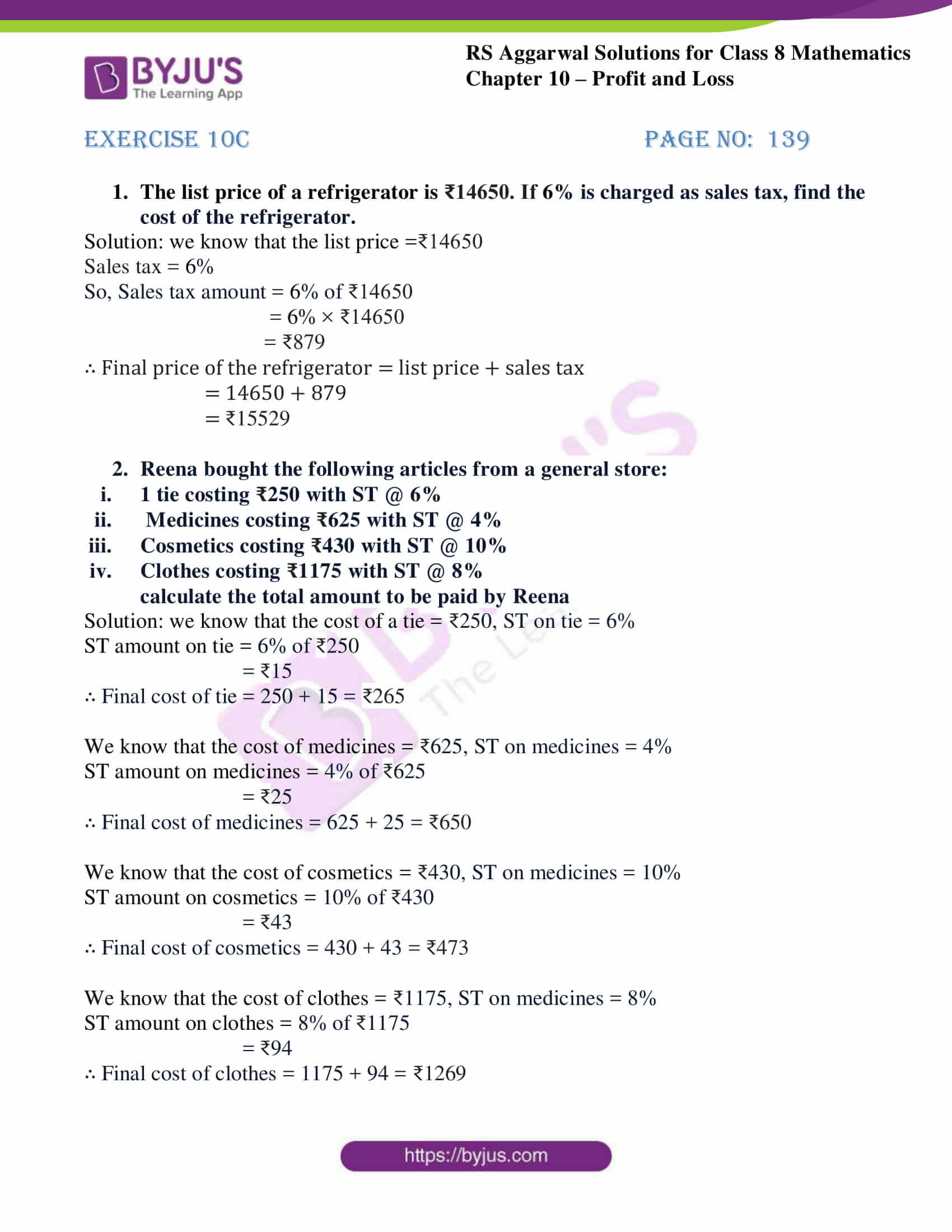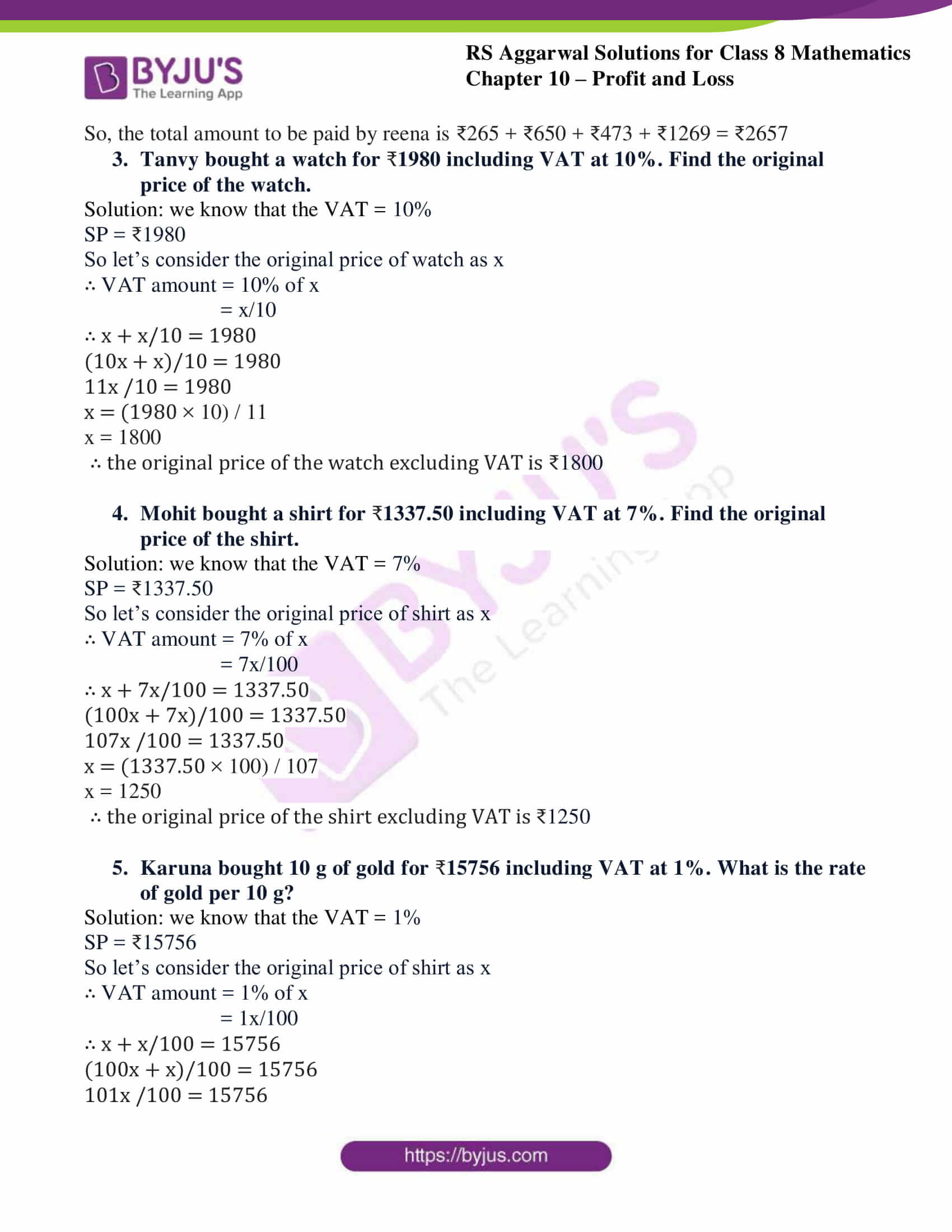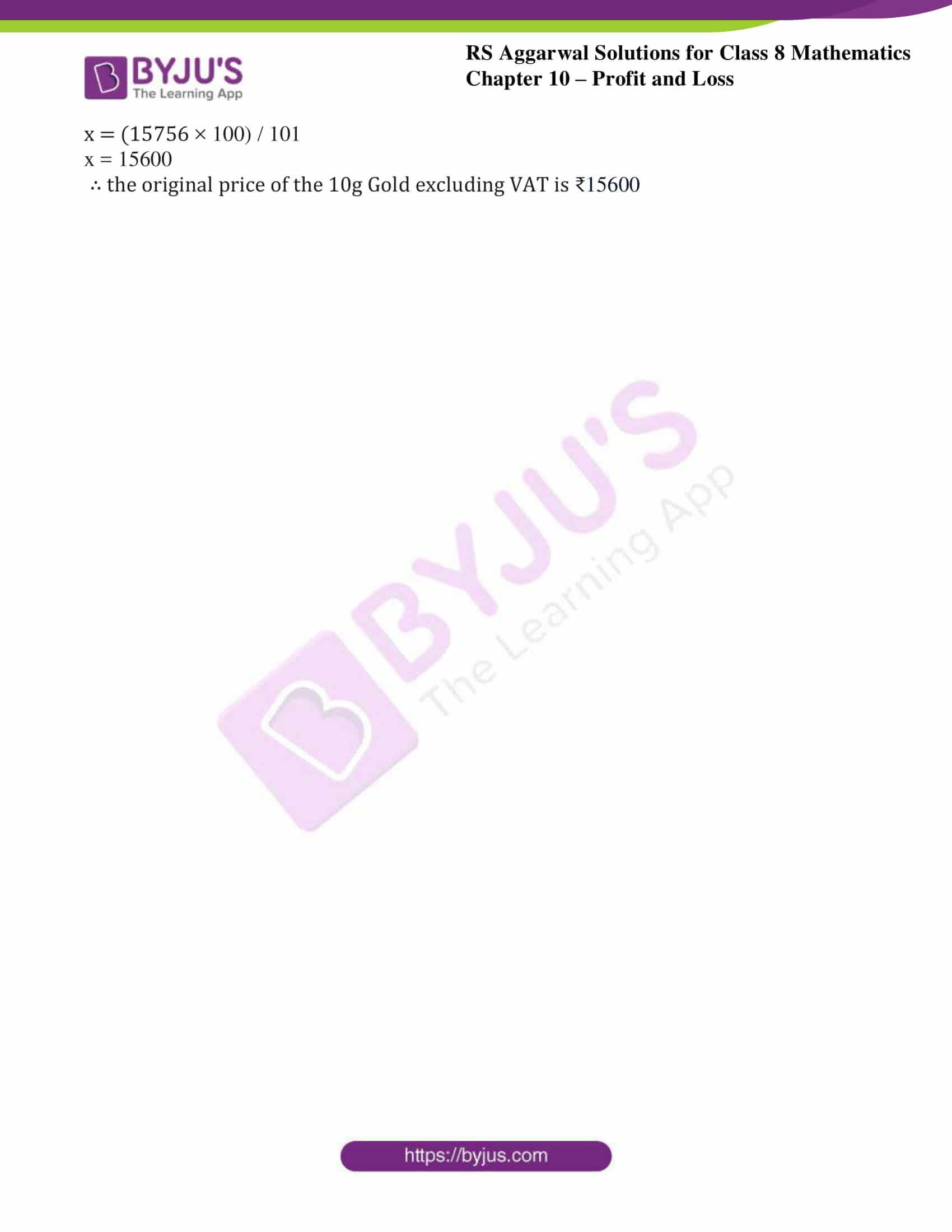# RS Aggarwal Solutions for Class 8 Maths Chapter 10 - Profit and Loss Exercise 10C

RS Aggarwal Solutions for Class 8 Maths Chapter 10- Exercise 10C, Profit and Loss links are provided below, students can refer and download them. Our expert team have solved the RS Aggarwal Solutions to help students for their exam preparation. In Exercise 10C of RS Aggarwal Class 8 Maths, we shall brief about sales tax (ST) and value-added tax (VAT).

## Download PDF of RS Aggarwal Solutions for Class 8 Maths Chapter 10- Profit and Loss Exercise 10C### Access Answers to RS Aggarwal Solutions for Class 8 Maths Chapter 10- Profit and Loss Exercise 10C

Q1. The list price of a refrigerator is ₹14650. If 6% is charged as sales tax, find the cost of the refrigerator.

Solution: we know that the list price =₹14650

Sales tax = 6%

So, Sales tax amount = 6% of ₹14650

= 6% × ₹14650

= ₹879

∴ Final price of the refrigerator = list price + sales tax

= 14650 + 879

= ₹15529

Q2. Reena bought the following articles from a general store:
i. 1 tie costing ₹250 with ST @ 6%
ii. Medicines costing ₹625 with ST @ 4%
iii. Cosmetics costing ₹430 with ST @ 10%
iv. Clothes costing ₹1175 with ST @ 8%
calculate the total amount to be paid by Reena

Solution: we know that the cost of a tie = ₹250, ST on tie = 6%

ST amount on tie = 6% of ₹250

= ₹15

∴ Final cost of tie = 250 + 15 = ₹265

We know that the cost of medicines = ₹625, ST on medicines = 4%

ST amount on medicines = 4% of ₹625

= ₹25

∴ Final cost of medicines = 625 + 25 = ₹650

We know that the cost of cosmetics = ₹430, ST on medicines = 10%

ST amount on cosmetics = 10% of ₹430

= ₹43

∴ Final cost of cosmetics = 430 + 43 = ₹473

We know that the cost of clothes = ₹1175, ST on medicines = 8%

ST amount on clothes = 8% of ₹1175

= ₹94

∴ Final cost of clothes = 1175 + 94 = ₹1269

So, the total amount to be paid by reena is ₹265 + ₹650 + ₹473 + ₹1269 = ₹2657

Q3. Tanvy bought a watch for 1980 including VAT at 10%. Find the original price of the watch.

Solution: we know that the VAT = 10%

SP = ₹1980

So let’s consider the original price of watch as x

∴ VAT amount = 10% of x

= x/10

∴ x + x/10 = 1980

(10x + x)/10 = 1980

11x /10 = 1980

x = (1980 × 10) / 11

x = 1800

∴ the original price of the watch excluding VAT is ₹1800

Q4. Mohit bought a shirt for 1337.50 including VAT at 7%. Find the original price of the shirt.

Solution: we know that the VAT = 7%

SP = ₹1337.50

So let’s consider the original price of shirt as x

∴ VAT amount = 7% of x

= 7x/100

∴ x + 7x/100 = 1337.50

(100x + 7x)/100 = 1337.50

107x /100 = 1337.50

x = (1337.50 × 100) / 107

x = 1250

∴ the original price of the shirt excluding VAT is ₹1250

Q5. Karuna bought 10 g of gold for 15756 including VAT at 1%. What is the rate of gold per 10 g?

Solution: we know that the VAT = 1%

SP = ₹15756

So let’s consider the original price of shirt as x

∴ VAT amount = 1% of x

= 1x/100

∴ x + x/100 = 15756

(100x + x)/100 = 15756

101x /100 = 15756

x = (15756 × 100) / 101

x = 15600

∴ the original price of the 10g Gold excluding VAT is ₹15600

### Access other Exercises of RS Aggarwal Solutions for Class 8 Maths Chapter 10- Profit and Loss

Exercise 10A Solutions 34 Questions

Exercise 10B Solutions 14Questions

Exercise 10D Solutions 21 Questions

## RS Aggarwal Solutions for Class 8 Maths Chapter 10- Profit and Loss Exercise 10C

Exercise 10C of RS Aggarwal Class 8, Profit and Loss. This exercise mainly deals with sales tax (ST)- ST is charged by the shopkeeper from the customer on the selling price of an item and is added to the value of the bill. Value-added tax (VAT)- These days, the prices of the articles include a tax called VAT. Different items carry VAT at different rates.

Students are suggested to practice the problems on a regular basis which will help them excel in their exams and increase their overall percentage. Practicing as many times as possible helps in building time management skills and also boosts the confidence level to achieve high marks.